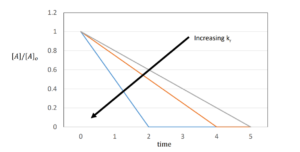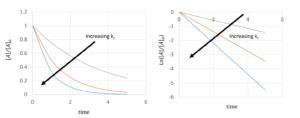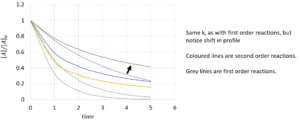# 14 Integrated Rate Laws

Learning Objectives

By the end of this section, you should be able to:

Determine the reaction order and rate constant from kinetic data using the linearized form of integrated rate laws

## Integrated Rate Laws

Rate laws are differential equations that can be integrated to find how the concentrations of reactants and products change with time. We can imagine a fairly complex system with multiple reactions described by

$𝑟_{1}=𝑘_{𝑟1}∗𝑓(𝐴,𝐵,...), 𝑟_{2}=𝑘_{𝑟2}∗𝑓(𝐴,𝐵,...), 𝑟_{3}, 𝑟_{4}...$

The concentration of all components can be described by equations such as $\frac{d[A]}{dt}=−r_{1}−2r_{2}...$ We need to integrate these to find concentrations at a given time.

Any of these rate laws can be solved numerically (you’ll learn about this in CHBE 230), but some simpler cases can also be solved analytically.

## Zeroth Order Reactions

A zeroth-order reaction is one whose rate is independent of concentration$^{}$; Say we have a reaction:

$A → B$

It’s differential rate law would be represented as:

$r = -\frac{d[A]}{dt} =k_{r}$

Integrating from t=0, when the system has a concentration of A as $[A]_{0}$, to some time t, when the system has a concentration represented by $[A]$

$\int_{[A]_{0}}^{[A]} \mathrm d[A] = \int_{t_{0}=0}^{t} \mathrm -k_{r}$

 $-[A]_{0}=-k_{r}*t$ or $[A]=[A]_{0}-k_{r}*t$## First-Order Reactions

Using a similar approach to the zeroth order reactions:

$r=-\frac{d[A]}{dt}=k_{r}[A]$

$\int_{[A]_{0}}^{[A]} \frac{d[A]}{[A]} = \int_{t_{0}=0}^{t} \mathrm-k_{r}$

 $ln[A]-ln[A]_{0}=-k_{r}*t$ or $[A]=[A]_{0}e^{-k_{r}*t}$Example: Half-life for First-order Reactions

Based on the equation we derived, what is an expression for the time taken for half of A to be consumed (half-life)?

$ln[A]-ln[A]_{0}=-k_{r}*t$

\begin{align*}
k_{r}* t_{1/2} & = -ln \frac{[A]}{[A]_{0}} \\
& = -ln \frac{\frac{1}{2}[A]_{0}}{[A]_{0}} \\
& =-ln(\frac{1}{2}) \\
& = ln(2)
\end{align*}

$t_{1/2}=\frac{ln(2)}{k_{r}}$

Note that this half-life does not depend on [A].

Practice: use $[A]=[A]_{0}e^{-k_{r}*t}$, you should get the same result.

Solution

\begin{align*}
[A] & = [A]_{0}*e^{-k_{r}t_{1/2}} \\
\frac{[A]}{[A]_{0}}& = e^{-k_{r}t_{1/2}} \\
\frac{1}{2} & = e^{-k_{r}t_{1/2}} \\
ln(\frac{1}{2}) & = -k_{r}*t_{1/2}
\end{align*}

$t_{1/2} =-\frac{ln(\frac{1}{2})}{k_{r}} = \frac{ln(2)}{k_{r}}$

## Second-Order Reactions

$r=-\frac{d[A]}{dt}=k_{r}[A]^2$

$\int_{[A]_{0}}^{[A]} \frac{d[A]}{[A]^2} = \int_{t_{0}=0}^{t} \mathrm-k_{r}$

 $\frac{1}{[A]}-\frac{1}{[A]_{0}}=k_{r}*t$ or $[A]=\frac{[A]_{0}}{1+k_{r}*t*[A]_{0}}$

Using this to find half life, we find that: $t_{1/2}=\frac{1}{k_{r}*[A]_{0}}$